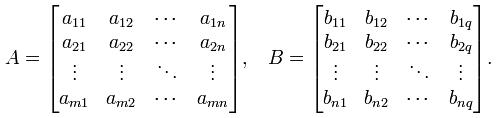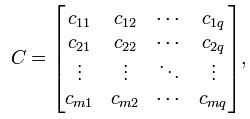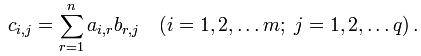Competitions

# Matrix multiplication

Given two square matrices A and B of dimensions m × n and n × q respectively:Then the matrix C of dimension m × q is called their product:where:The operation of multiplication of two matrices is feasible only if the number of columns in the first factor equals to the number of rows in the second. In this case we say that the shape of the matrices is consistent.

Given two matrices A and B. Find their product.

#### Input

The first line contains two positive integers na and ma - the dimensions of matrix A. Each of the next na rows contains ma numbers - the elements aij of matrix A. In (na + 2)-nd row two positive integers nb and mb are given - the dimensions of matrix B. In the following nb lines mb numbers are given - the elements bij of matrix B. Dimensions of the matrices do not exceed 100 × 100, all integers do not exceed 100 by absolute value.

#### Output

Print in the first line the dimensions of the resulting matrix C: nс and mc. In the next nс rows print space separated mc numbers - the corresponding elements cij of matrix C. If you cannot multiply matrixs in the first and only line of output -1.

Time limit 1 second
Memory limit 128 MiB
Input example #1
2 3
1 3 4
5 -2 3
3 3
1 3 2
2 1 3
0 -1 1

Output example #1
2 3
7 2 15
1 10 7

Input example #2
2 3
10 12 31
23 11 17
3 4
0 2 1 4
3 9 2 4
-5 4 2 5

Output example #2
2 4
-119 252 96 243
-52 213 79 221